# Reflection

A mappingof an-dimensional simply-connected space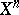of constant curvature (i.e. of a Euclidean or affine space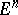, a sphere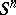or a hyperbolic (Lobachevskii) space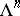) the set of fixed pointsof which is an-dimensional hyperplane. The setis called the mirror of the mapping; in other words,is a reflection in. Every reflection is uniquely defined by its mirror. The order (period) of a reflection in the group of all motions ofis equal to 2, i.e..

The Euclidean or affine spacecan be identified with the vector space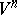of its parallel translations. The mappingis then a linear orthogonal transformation ofwith matrix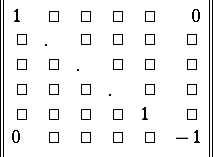in a certain orthonormal basis, and conversely, every orthogonal transformation ofwith this matrix in a certain orthonormal basis is a reflection in. More generally, a linear transformationof an arbitrary vector spaceover a field, of characteristic other than 2, is called a linear reflection if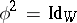and if the rank of the transformation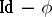is equal to. In this case, the subspace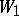of fixed vectors relative tohas codimensionin, the subspace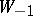of eigenvectors with eigenvaluehas dimensionand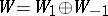. Ifis a linear form onsuch that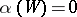when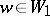, and if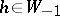is an element such that, thenis defined by the formulaThe description of a reflection in an arbitrary simply-connected spaceof constant curvature can be reduced to the description of linear reflections in the following way. Every such spacecan be imbedded as a hypersurface in a real-dimensional vector spacein such a way that the motions ofcan be extended to linear transformations of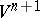. Moreover, in a suitable coordinate system inthe equations of the hypersurface can be written in the following way: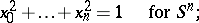Every hypersurface in, given this imbedding, is the intersection withof a certain-dimensional subspace in, and every reflection inis induced by a linear reflection in.

If, in the definition of a linear reflection, the requirement thatis dropped, then the more general concept of a pseudo-reflection is obtained. Ifis the field of complex numbers andis a pseudo-reflection of finite order (not necessarily equal to 2), thenis called a unitary reflection. Every biholomorphic automorphism of finite order of a bounded symmetric domain in a complex space the set of fixed points of which has a complex codimension 1 is also called a unitary reflection.